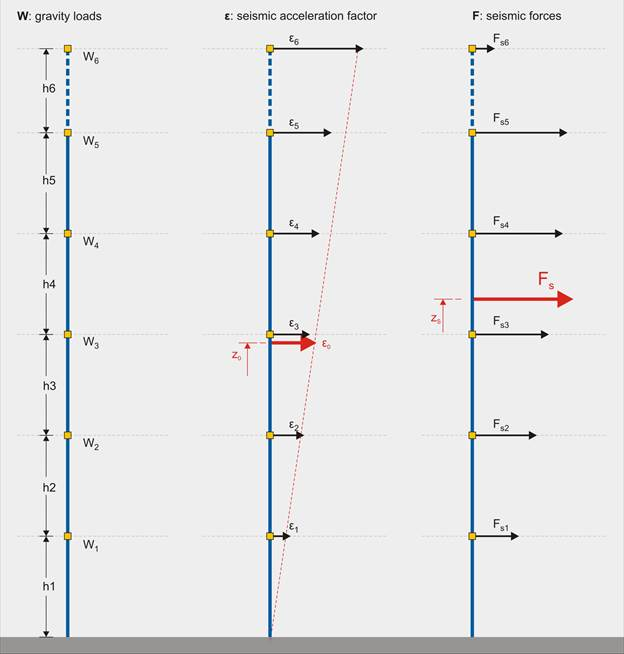Introduction >
The structural frame
The construction
The reinforcement I
The reinforcement II
Quantity/Cost estimation
Detailing drawingsIntroduction >
Wind and Seismic Forces >
Structural model and Analysis
Modelling slabs
Slabs
Seismic behavour of frames
Appendix A
Appendix B
Appendix C
Appendix DIntroduction >
Materials
To be continued >The effect that a seismic action will have in a structure is determined by regarding an earthquake with horizontal design ground seismic acceleration equal to A=α*g

In the duration of an earthquake, a horizontal seismic force H is applied in every mass M that has gravity load W. The H force is equal to a percentage ε of the W force. ε value usually varies between 0.00 and 0.50 while in a very intense seismic action it may rise above 1.00.

## Seismic acceleration distributionIn general terms, the distribution of seismic accelerations has the form of a triangle.

The structure shown above belongs to the Seismic Zone Z1 (seismic ground acceleration equal to 0.16), the mean value of the design seismic acceleration is around 0.12g (ε0=0.12) and the resultant seismic force Fs is around 1400 kN. The theoretical height that this force is applied to, is around the 2/3 of the structure’s total height.

## Comparison of wind forces Fw and seismic forces FsComparing the wind and the seismic forces applied to that structure we realize that the wind effect upon the structure is at least four times smaller than the seismic effect

In the same structure, when placed in a geographical region with intense winds, the mean value of the wind pressure is around 1.50 kN/m² and the resultant force around 400 kN. The total service load applied is 12.000 kN. Therefore the wind force is equal to 0.03% of the vertical loads and its theoretical application area is in the middle of the structure’s height.